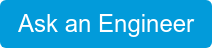×

# Humidity Definitions - Part 2

Relative Humidity (RH)
Relative humidity (RH) is the ratio of the actual vapor pressure (Pw) in the gas mixture to the saturation vapor pressure (Pws), with respect to water, at the prevailing dry bulb temperature. Relative humidity is expressed as a percentage.Example 1.
If the dew point = 10°C and the dry bulb = 25°C:If the frost point = -45°C and the dry bulb = -40°C:Example 2.
If the dew point = 50°F and the dry bulb = 90°F:If the frost point = -50°F and the dry bulb = -40°F:NOTE: RH is arbitrarily defined with respect to water even though it seems that it should be with respect to ice at -40°C (-40°F).

Parts Per Million by Volume (PPMv)
Parts per million by volume (PPMv) is the ratio of the partial pressure of water vapor to the partial pressure of the dry gas.

Example 1.
If the frost point = -60°C and system total pressure (Pm) is 1013 mb:Example 2. (English Units)
If frost point = -70°F and Pm is 29.92 inHg:### Categories